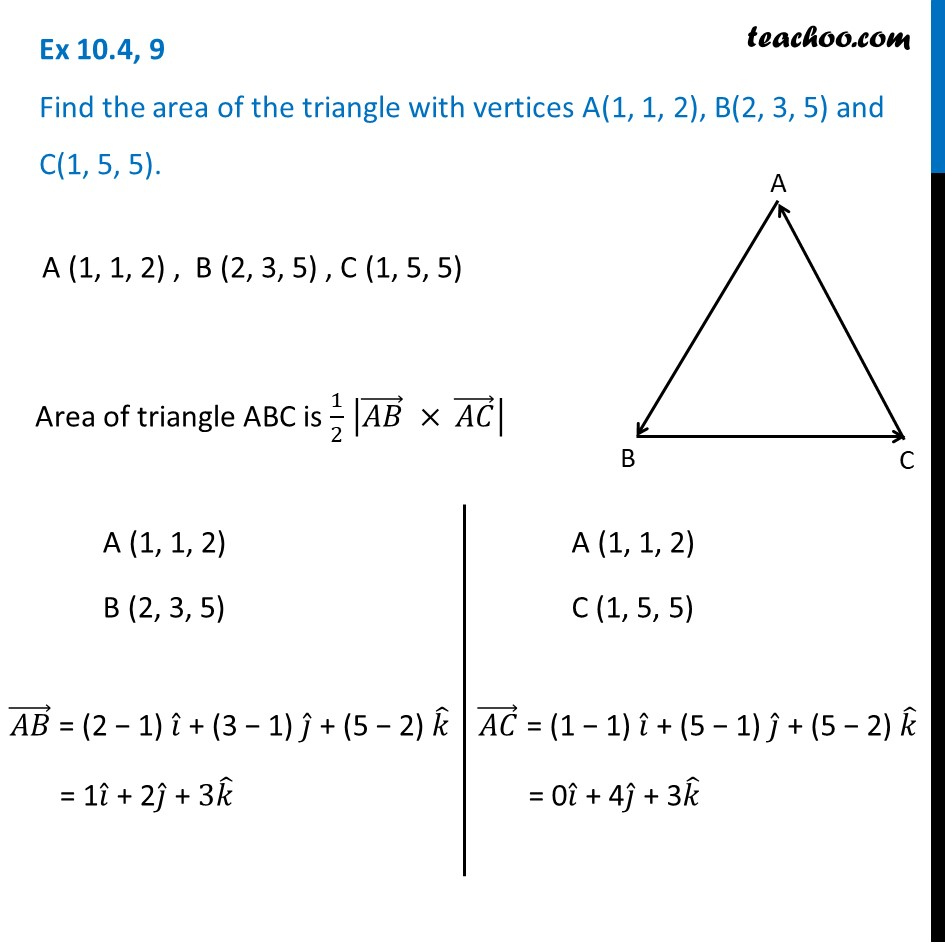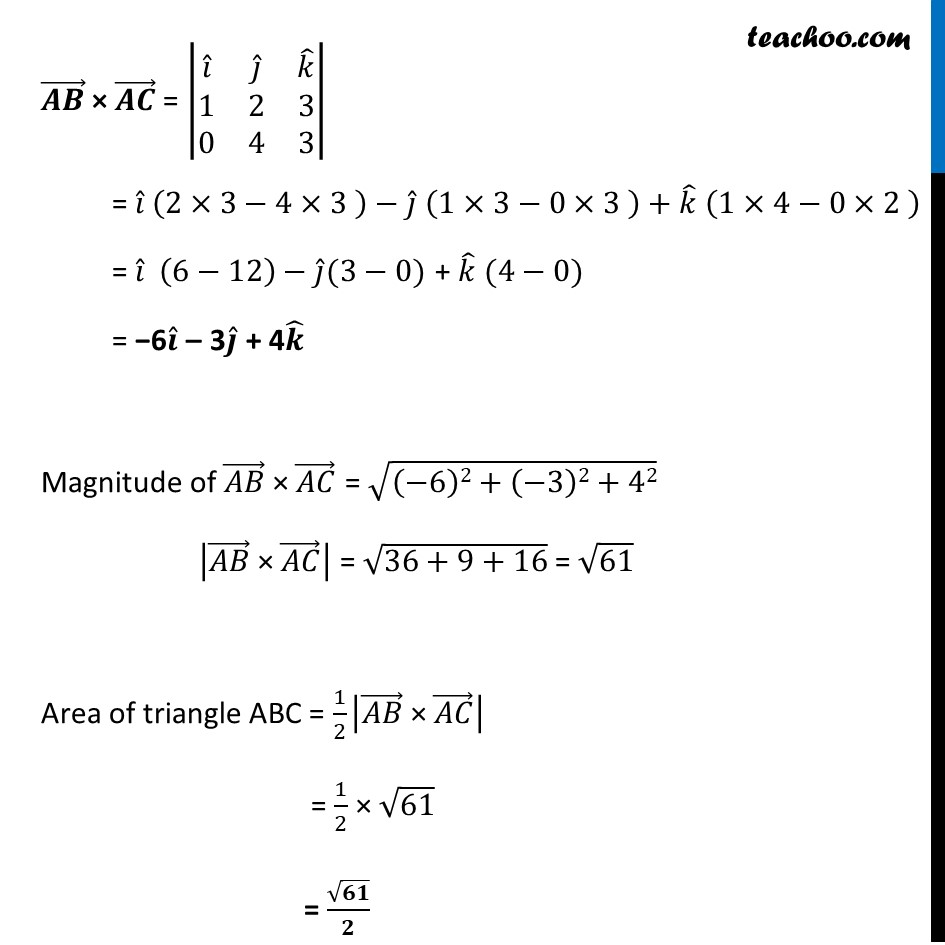Ex 10.4

Chapter 10 Class 12 Vector Algebra
Serial order wiseLearn in your speed, with individual attention - Teachoo Maths 1-on-1 Class

### Transcript

Ex 10.4, 9 Find the area of the triangle with vertices A(1, 1, 2), B(2, 3, 5) and C(1, 5, 5). A (1, 1, 2) , B (2, 3, 5) , C (1, 5, 5) Area of triangle ABC is 1/2 |(𝐴𝐵) ⃗ × (𝐴𝐶) ⃗ | A (1, 1, 2) B (2, 3, 5) (𝐴𝐵) ⃗ = (2 − 1) 𝑖 ̂ + (3 − 1) 𝑗 ̂ + (5 − 2) 𝑘 ̂ = 1𝑖 ̂ + 2𝑗 ̂ + 3𝑘 ̂ A (1, 1, 2) C (1, 5, 5) (𝐴𝐶) ⃗ = (1 − 1) 𝑖 ̂ + (5 − 1) 𝑗 ̂ + (5 − 2) 𝑘 ̂ = 0𝑖 ̂ + 4𝑗 ̂ + 3𝑘 ̂ (𝑨𝑩) ⃗ × (𝑨𝑪) ⃗ = |■8(𝑖 ̂&𝑗 ̂&𝑘 ̂@1&2&[email protected]&4&3)| = 𝑖 ̂ (2×3−4×3 )−𝑗 ̂ (1×3−0×3 )+𝑘 ̂ (1×4−0×2 ) = 𝑖 ̂ (6−12)−𝑗 ̂(3−0) + 𝑘 ̂ (4−0) = −6𝒊 ̂ – 3𝒋 ̂ + 4𝒌 ̂ Magnitude of (𝐴𝐵) ⃗ × (𝐴𝐶) ⃗ = √((−6)2+(−3)2+42) |(𝐴𝐵) ⃗" × " (𝐴𝐶) ⃗ | = √(36+9+16) = √61 Area of triangle ABC = 1/2 |(𝐴𝐵) ⃗" × " (𝐴𝐶) ⃗ | = 1/2 × √61 = √𝟔𝟏/𝟐